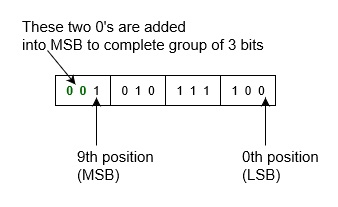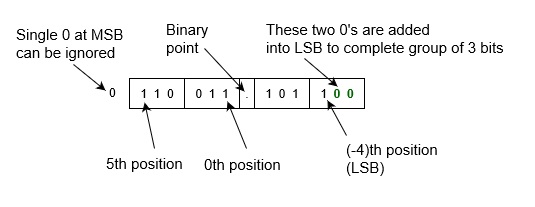# How to Convert Binary to Octal?

Binary is the simplest kind of number system that uses only two digits of 0 and 1 (i.e. value of base 2). Since digital electronics have only these two states (either 0 or 1), so binary number is most preferred in modern computer engineer, networking and communication specialists, and other professionals.

Whereas Octal number is one of the number systems which has value is 10 and it has only 8 symbols: 0, 1, 2, 3, 4, 5, 6, and 7.

## Conversion from Binary to Octal number system

Octal number system provides convenient way of converting large binary numbers into more compact and smaller groups. There are various ways to convert a binary number into octal number. You can convert using direct methods or indirect methods. First, you need to convert a binary into other base system (e.g., into decimal, or into hexadecimal). Then you need to convert it octal number.

Most Significant Bit (MSB)Octal PointLeast Significant Bit (LSB)
8281808-18-28-3
64811/81/641/512

Since number numbers are type of positional number system. That means weight of the positions from right to left are as 80, 81, 82, 83 and so onfor the integer part and weight of the positions from left to right are as 8-1, 8-2, 8-3and so on.for the fractional part.

Example − Convert binary number 10010110 into octal number.

First convert this into decimal number
= (10010110)2
= 1x27+0x26+0x25+1x24+0x23+1x22+1x21+0x20
= 128+0+0+16+0+4+2+0
= (150)10
Then, convert it into octal number
= (150)10
= 2x82+2x81+6x80
= (226)8 which is answer.

However, there is also a direct method to convert a binary number into octal number − grouping which is explained as following below.

## Using Grouping

Since, there are only 8 digits (from 0 to 7) in octal number system, so we can represent any digit of octal number system using only 3 bit as following below.

Octal Digit ValueBinary Equivalent
0000
1001
2010
3011
4100
5101
6110
7111

So, if you make each group of 3 bit of binary input number, then replace each group of binary number from its equivalent octal digits. That will be octal number of given number number. Note that you can add any number of 0’s in leftmost bit (or in most significant bit) for integer part and add any number of 0’s in rightmost bit (or in least significant bit) for fraction part for completing the group of 3 bit, this does not change value of input binary number.

So, these are following steps to convert a binary number into octal number.

• Take binary number

• Divide the binary digits into groups of three (starting from right) for integer part and start from left for fraction part.

• Convert each group of three binary digits to one octal digit.

This is simple algorithm where you have to grouped binary number and replace their equivalent octal digit.

Example-1 − Convert binary number 1010111100 into octal number. Since there is no binary point here and no fractional part. So,Therefore, Binary to octal is.

= (1010111100)2
= (001 010 111 100)2
= (1 2 7 4)8
= (1274)8

Example-2 Convert binary number 0110 011.1011 into octal number. Since there is binary point here and fractional part. So,Therefore, Binary to octal is.

= (0110 011.1011)2
= (0 110 011 . 101 1)2
= (110 011 . 101 100)2
= (6 3 . 5 4)8
= (63.54)8

These are above simple conversions binary number to octal number.